Get Started »

# Trigonometry

For basic trig functions, use standard abbreviations (with the first letter capitalized):

 In:=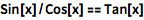⨯ `Sin[x]/Cos[x] == Tan[x]`
 Out=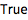In:=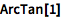⨯ `ArcTan`
 Out=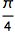Use Pi when working with radian expressions:

(Type ESCpiESC for the `π` character.)
 In:=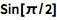⨯ `Sin[\[Pi]/2]`
 Out=Or type ESCdegESC for the built-in Degree symbol:

 In:=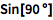⨯ `Sin[90 \[Degree]]`
 Out=Automatically expand (or reduce) trig expressions using identities:

 In:=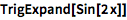⨯ `TrigExpand[Sin[2 x]]`
 Out=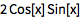Factor trig polynomials:

 In:=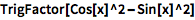⨯ `TrigFactor[Cos[x]^2 - Sin[x]^2]`
 Out=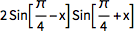Functions like Solve work as well:

 In:=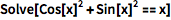⨯ `Solve[Cos[x]^2 + Sin[x]^2 == x]`
 Out=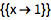Specify a domain for solutions:

 In:=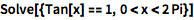⨯ `Solve[{Tan[x] == 1, 0 < x < 2 Pi}]`
 Out=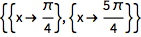QUICK REFERENCE: Trigonometric Functions `»`